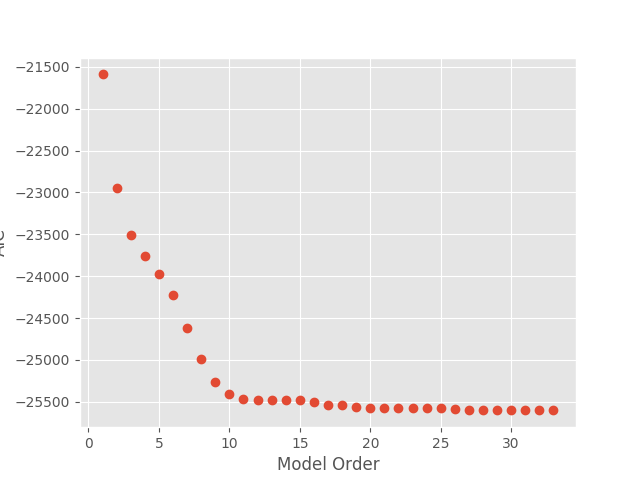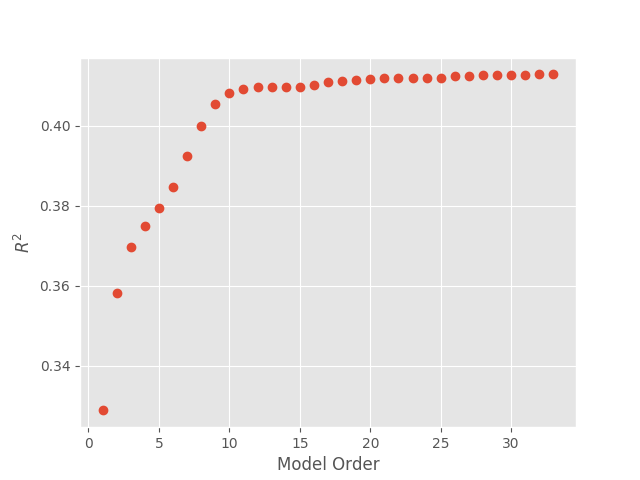# Tutorial 4 - Exploring model order¶

In this tutorial, we will examine how to look at different model orders in order to determine the most appropriate model.

The first section of this tutorial should be familiar to you from previous tutorials. We start by loading in some data:

from os.path import join

import h5py

import numpy as np
import matplotlib.pyplot as plt

from sails import find_example_path, VieiraMorfLinearModel

plt.style.use('ggplot')

ex_dir = find_example_path()

sample_rate = 2034.51 / 24
nyq = sample_rate / 2.

freq_vect = np.linspace(0, nyq, 64)

X = h5py.File(join(ex_dir, 'meg_single.hdf5'), 'r')['X'][...]


We will use the AIC values to examine how appropriate our model is. We therefore set up an empty list to store our AIC values in and fit a model for a range of different orders. We can then plot up the values of AIC.

AICs = []

orders = []

for delays in range(2, 35):
delay_vect = np.arange(delays)
m = VieiraMorfLinearModel.fit_model(X, delay_vect)
diag = m.compute_diagnostics(X)
orders.append(m.order)
AICs.append(diag.AIC)

f = plt.figure()

plt.plot(orders, AICs, 'o');

plt.xlabel('Model Order')
plt.ylabel('AIC')

f.show()The same principle can be used for any of the other measures such as BIC and R-squared. You should always be aware that the change the number of parameters in the model for different orders means that certain measures (such as R-squared) will always increase for increasing model order.

R_squares = []

orders = []

for delays in range(2, 35):
delay_vect = np.arange(delays)
m = VieiraMorfLinearModel.fit_model(X, delay_vect)
diag = m.compute_diagnostics(X)
orders.append(m.order)
R_squares.append(diag.R_square)

f2 = plt.figure()

plt.plot(orders, R_squares, 'o');

plt.xlabel('Model Order')
plt.ylabel('$R^2$')

f2.show()